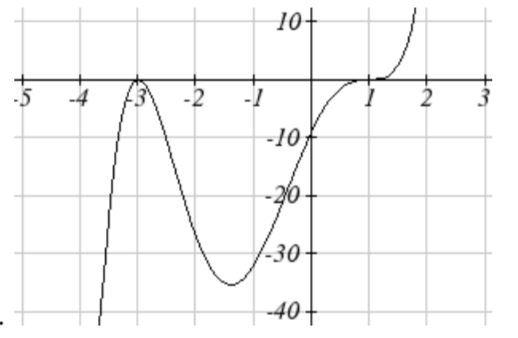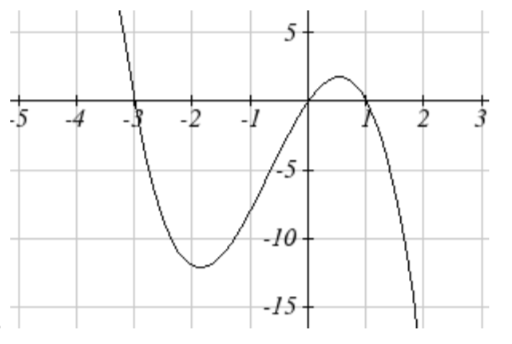$$\newcommand{\id}{\mathrm{id}}$$ $$\newcommand{\Span}{\mathrm{span}}$$ $$\newcommand{\kernel}{\mathrm{null}\,}$$ $$\newcommand{\range}{\mathrm{range}\,}$$ $$\newcommand{\RealPart}{\mathrm{Re}}$$ $$\newcommand{\ImaginaryPart}{\mathrm{Im}}$$ $$\newcommand{\Argument}{\mathrm{Arg}}$$ $$\newcommand{\norm}{\| #1 \|}$$ $$\newcommand{\inner}{\langle #1, #2 \rangle}$$ $$\newcommand{\Span}{\mathrm{span}}$$

# 3.3.3E: Graphs of Polynomial Functions (Exercises)

$$\newcommand{\vecs}{\overset { \rightharpoonup} {\mathbf{#1}} }$$ $$\newcommand{\vecd}{\overset{-\!-\!\rightharpoonup}{\vphantom{a}\smash {#1}}}$$$$\newcommand{\id}{\mathrm{id}}$$ $$\newcommand{\Span}{\mathrm{span}}$$ $$\newcommand{\kernel}{\mathrm{null}\,}$$ $$\newcommand{\range}{\mathrm{range}\,}$$ $$\newcommand{\RealPart}{\mathrm{Re}}$$ $$\newcommand{\ImaginaryPart}{\mathrm{Im}}$$ $$\newcommand{\Argument}{\mathrm{Arg}}$$ $$\newcommand{\norm}{\| #1 \|}$$ $$\newcommand{\inner}{\langle #1, #2 \rangle}$$ $$\newcommand{\Span}{\mathrm{span}}$$ $$\newcommand{\id}{\mathrm{id}}$$ $$\newcommand{\Span}{\mathrm{span}}$$ $$\newcommand{\kernel}{\mathrm{null}\,}$$ $$\newcommand{\range}{\mathrm{range}\,}$$ $$\newcommand{\RealPart}{\mathrm{Re}}$$ $$\newcommand{\ImaginaryPart}{\mathrm{Im}}$$ $$\newcommand{\Argument}{\mathrm{Arg}}$$ $$\newcommand{\norm}{\| #1 \|}$$ $$\newcommand{\inner}{\langle #1, #2 \rangle}$$ $$\newcommand{\Span}{\mathrm{span}}$$

section 3.3 exercise

Find the $$C$$ and $$t$$ intercepts of each function.

1. $$C\left(t\right)=2\left(t-4\right)\left(t+1\right)(t-6)$$

2. $$C\left(t\right)=3\left(t+2\right)\left(t-3\right)(t+5)$$

3. $$C\left(t\right)=4t\left(t-2\right)^{2} (t+1)$$

4. $$C\left(t\right)=2t\left(t-3\right)\left(t+1\right)^{2}$$

5. $$C\left(t\right)=2t^{4} -8t^{3} +6t^{2}$$

6. $$C\left(t\right)=4t^{4} +12t^{3} -40t^{2}$$

Use your calculator or other graphing technology to solve graphically for the zeros of the function.

7. $$f\left(x\right)=x^{3} -7x^{2} +4x+30$$

8. $$g\left(x\right)=x^{3} -6x^{2} +x+28$$

Find the long run behavior of each function as $$t \to \infty$$ and $$t \to -\infty$$

9. $$h\left(t\right)=3\left(t-5\right)^{3} \left(t-3\right)^{3} (t-2)$$

10. $$k\left(t\right)=2\left(t-3\right)^{2} \left(t+1\right)^{3} (t+2)$$

11. $$p\left(t\right)=-2t\left(t-1\right)\left(3-t\right)^{2}$$

12. $$q\left(t\right)=-4t\left(2-t\right)\left(t+1\right)^{3}$$

Sketch a graph of each equation.

13. $$f\left(x\right)=\left(x+3\right)^{2} (x-2)$$

14. $$g\left(x\right)=\left(x+4\right)\left(x-1\right)^{2}$$

15. $$h\left(x\right)=\left(x-1\right)^{3} \left(x+3\right)^{2}$$

16. $$k\left(x\right)=\left(x-3\right)^{3} \left(x-2\right)^{2}$$

17. $$m\left(x\right)=-2x\left(x-1\right)(x+3)$$

18. $$n\left(x\right)=-3x\left(x+2\right)(x-4)$$

Solve each inequality.

19. $$\left(x-3\right)\left(x-2\right)^{2} >0$$

20. $$\left(x-5\right)\left(x+1\right)^{2} >0$$

21. $$\left(x-1\right)\left(x+2\right)\left(x-3\right)<0$$

22. $$\left(x-4\right)\left(x+3\right)\left(x+6\right)<0$$

Find the domain of each function.

23. $$f\left(x\right)=\sqrt{-42+19x-2x^{2} }$$

24. $$g\left(x\right)=\sqrt{28-17x-3x^{2} }$$

25. $$h\left(x\right)=\sqrt{4-5x+x^{2} }$$

26. $$k\left(x\right)=\sqrt{2+7x+3x^{2} }$$

27. $$n\left(x\right)=\sqrt{\left(x-3\right)\left(x+2\right)^{2} }$$

28. $$m\left(x\right)=\sqrt{\left(x-1\right)^{2} (x+3)}$$

29. $$p\left(t\right)=\dfrac{1}{t^{2} +2t-8}$$

30. $$q\left(t\right)=\dfrac{4}{x^{2} -4x-5}$$

Write an equation for a polynomial the given features.

31. Degree 3. Zeros at $$x$$ = -2, $$x$$ = 1, and $$x$$ = 3. Vertical intercept at (0, -4)

32. Degree 3. Zeros at $$x$$ = -5, $$x$$ = -2, and $$x$$ = 1. Vertical intercept at (0, 6)

33. Degree 5. Roots of multiplicity 2 at $$x$$ = 3 and $$x$$ = 1, and a root of multiplicity 1 at $$x$$ = -3. Vertical intercept at (0, 9)

34. Degree 4. Root of multiplicity 2 at $$x$$ = 4, and a roots of multiplicity 1 at $$x$$ = 1 and $$x$$ = -2. Vertical intercept at (0, -3)

35. Degree 5. Double zero at $$x$$ = 1, and triple zero at $$x$$ = 3. Passes through the point (2, 15)

36. Degree 5. Single zero at $$x$$ = -2 and $$x$$ = 3, and triple zero at $$x$$ = 1. Passes through the point (2, 4)

Write a formula for each polynomial function graphed.

37.38.39.40.41.42.43.44.Write a formula for each polynomial function graphed.

45.46.47.48.49.50.51. A rectangle is inscribed with its base on the $$x$$ axis and its upper corners on the parabola $$y=5-x^{2}$$. What are the dimensions of such a rectangle that has the greatest possible area?

52. A rectangle is inscribed with its base on the $$x$$ axis and its upper corners on the curve $$y=16-x^{4}$$. What are the dimensions of such a rectangle that has the greatest possible area?

 $$C(t)$$ $$C$$, intercepts $$t$$, intercepts 1. (0, 48) (4, 0), (-1, 0), (6, 0) 3. (0, 0) (0, 0), (2, 0), (-1, 0) 5. (0, 0) (0, 0), (1, 0), (3, 0)

7. (-1.646, 0) (3.646, 0) (5, 0)

9. As $$t \to \infty$$, $$h(t) \to \infty$$ $$t \to -\infty$$, $$h(t) \to -\infty$$

11. As $$t \to \infty$$, $$p(t) \to -\infty$$ $$t \to -\infty$$, $$p(t) \to -\infty$$

13.15.17.19. $$(3, \infty)$$

21. $$(-\infty, -2) \cup (1, 3)$$

23. [3, 5, 6]

25. $$(-\infty, 1] \cup [4, \infty)$$

27. $$[-2, -2] \cup [3, \infty)$$

29. $$(-\infty, -4) \cup (-4, 2) \cup (2, \infty)$$

31. $$y = -\dfrac{2}{3} (x + 2) (x - 1) (x - 3)$$

33. $$y = \dfrac{1}{3} (x - 1)^2 (x - 3)^2 (x + 3)$$

35. $$y = -15(x - 1)^2 (x - 3)^2$$

37. $$y = \dfrac{1}{2} (x + 2)(x - 1) (x - 3)$$

39. $$y = -(x + 1)^2 (x - 2)$$

41. $$y = -\dfrac{1}{24} (x + 3)(x + 2) (x - 2) (x - 4)$$

43. $$y = \dfrac{1}{24} (x + 4) (x + 2) (x - 3)^2$$

45. $$y = \dfrac{1}{12} (x + 2)^2 (x - 3)^2$$

47. $$y = \dfrac{1}{6} (x + 3) (x + 2) (x - 1)^3$$

49. $$y = -\dfrac{1}{16} (x + 3)(x + 1) (x - 2)^2 (x - 4)$$

51. Base 2.58, Height 3.336